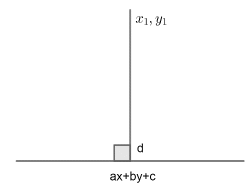QuestionAnswers

# If $4{{l}^{2}}-5{{m}^{2}}+6l+1=0,$ then show that line $lx+my+1=0$ touches a fixed circle. Find the equation of the circle.Verified
158.1k+ views
Hint: Assume a circle and apply the condition to tangency on the given line to this assumed circle. Compare this equation with the equation given in the question.

Let us consider a circle having its equation ${{\left( x-h \right)}^{2}}+{{\left( y-k \right)}^{2}}={{a}^{2}}$. The circle is in its standard form. So its centre is $\left( h,k \right)$ and radius $=a$.
Since line $lx+my+1=0$ touches this circle, the perpendicular distance from centre of the circle ${{\left( x-h \right)}^{2}}+{{\left( y-k \right)}^{2}}={{a}^{2}}$ to the line $lx+my+1=0$is equal to radius.

To find the perpendicular distance from a point $\left( {{x}_{1}},{{y}_{1}} \right)$ to a line $ax+by+c=0$, the formula to find this perpendicular distance $'d'$ is given by$d=\dfrac{\left| a{{x}_{1}}+b{{y}_{1}}+c \right|}{\sqrt{{{a}^{2}}+{{b}^{2}}}}........\left( i \right)$
Using formula $\left( i \right)$ to find perpendicular distance by substituting $a=l\text{,}b=m,c=1\text{, }{{x}_{1}}=h,{{y}_{1}}=k$ in equation $\left( i \right)$, we get 🡪
$d=\dfrac{\left| lh+mk+1 \right|}{\sqrt{{{l}^{2}}+{{m}^{2}}}}$
As explained in the above paragraph, this distance should be equal to the radius of the circle.
So, $d=a$
Since we have found that $d=\dfrac{\left| lh+mk+1 \right|}{\sqrt{{{l}^{2}}+{{m}^{2}}}}$, hence 🡪
$\dfrac{\left| lh+mk+1 \right|}{\sqrt{{{l}^{2}}+{{m}^{2}}}}=a$

Squaring both sides, we get 🡪
$\dfrac{{{\left( lh+mk+1 \right)}^{2}}}{{{l}^{2}}+{{m}^{2}}}={{a}^{2}}$
$\Rightarrow {{l}^{2}}{{h}^{2}}+{{m}^{2}}{{k}^{2}}+1+2lh+2mk+2lhmk={{a}^{2}}\left( {{l}^{2}}+{{m}^{2}} \right)$
$\Rightarrow {{l}^{2}}{{h}^{2}}+{{m}^{2}}{{k}^{2}}+1+2lh+2mk+2lhmk={{a}^{2}}{{l}^{2}}+{{a}^{2}}{{m}^{2}}$
$\Rightarrow \left( {{l}^{2}}{{h}^{2}}-{{a}^{2}}{{l}^{2}} \right)+\left( {{m}^{2}}{{k}^{2}}-{{a}^{2}}{{m}^{2}} \right)+1+2lh+2mk+2lhmk=$
$\Rightarrow {{l}^{2}}\left( {{h}^{2}}-{{a}^{2}} \right)+{{m}^{2}}\left( {{k}^{2}}-{{a}^{2}} \right)+2hklm+2hl+2km+1=0.............\left( ii \right)$
It is given in question;
$4{{l}^{2}}-5{{m}^{2}}+6l+1=0......\left( iii \right)$

So we have to compare the equations $\left( ii \right)$ and $\left( iii \right)$. To compare two equations, we have to equate the ratio of coefficient of same and common variable in the two equations.

I)Equating ratio of coefficients of $l$ in equations $\left( ii \right)$ and $\left( iii \right)$ to the ratio of constant terms in equations $\left( ii \right)$ and $\left( iii \right)$ 🡪
\begin{align} & \dfrac{2h}{6}=\dfrac{1}{1} \\ & \Rightarrow h=\dfrac{6}{2} \\ & \Rightarrow h=3 \\ \end{align}
II) Equating ratio of coefficients of $m$ in equations $\left( ii \right)$ and $\left( iii \right)$ to the ratio
of constant terms in equations $\left( ii \right)$ and $\left( iii \right)$ 🡪\begin{align} & \left( \dfrac{2k}{0} \right)=\dfrac{1}{1} \\ & \Rightarrow k=0 \\ \end{align}

III)Equating ratio of coefficients of $'{{l}^{2}}'$ in equations $\left( ii \right)$ and $\left( iii \right)$ to the ratio of constant terms in equations $\left( ii \right)$ and $\left( iii \right)$ 🡪
\begin{align} & \dfrac{{{h}^{2}}-{{a}^{2}}}{4}=\dfrac{1}{1} \\ & \Rightarrow {{h}^{2}}-{{a}^{2}}=4 \\ & \Rightarrow {{\left( 3 \right)}^{2}}-{{a}^{2}}=4 \\ & \Rightarrow {{a}^{2}}=9-4 \\ & \Rightarrow {{a}^{2}}=5 \\ & \Rightarrow a=\sqrt{5} \\ \end{align}
Since there exists a single value of $h,\text{ }k,\text{ }a$, the circle is a fixed circle. Also, substituting
$h=3,k=0,a=\sqrt{5}$ in the assumed circle’s equation i.e. ${{\left( x-h \right)}^{2}}+{{\left( y-k \right)}^{2}}={{a}^{2}}$, we get the equation of circle 🡪
${{\left( x-3 \right)}^{2}}+{{\left( y-0 \right)}^{2}}={{\sqrt{5}}^{2}}$
${{\left( x-3 \right)}^{2}}+{{y}^{2}}=5$

Note: There is a possibility of committing mistakes while equating the coefficient ratio. So, in order to avoid this mistake, first collect all the terms of which we have to find the coefficient and then take the coefficient out of that term.